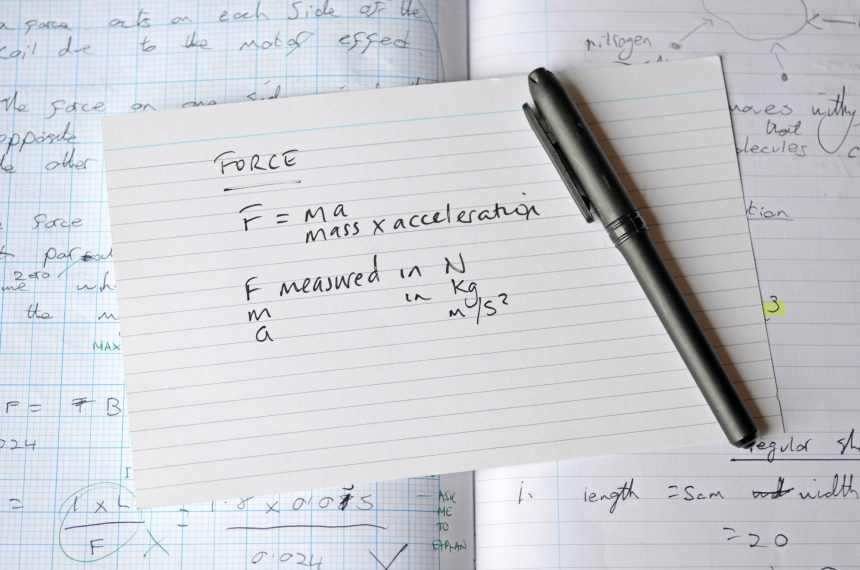The majority of students studying A Level Physics in the UK also study at least one maths A Level. The advantages of doing so benefit both subjects and include content overlap (particularly in mechanics) and increased dexterity in terms of equation manipulation and calculation work over the two years of each course. Also, by the time students encounter natural logarithms and exponentials in the topics of capacitance and radioactivity in physics, they will usually have covered these aspects in maths already.

However, there are students who take physics without maths. This can be for a myriad of reasons, from the polymath studying a wide breadth of A Level subjects, to the student who struggles with maths.

For the latter student, it is vital that they are supported in enhancing key GCSE Maths skills, to enable them to flourish in their physics studies. After all, 40% of exam marks across the question papers will assess mathematical skills at higher tier GCSE level. It should be noted, however, that other subject choices can be beneficial from a maths perspective. These include chemistry, biology, computing, and economics. Each of these A Levels will provide opportunities to develop maths skills.From a physics perspective, as a starting point, exam boards helpfully provide bridging material for teachers to use: here is an example from AQA. There are plenty of books available, in a similar vein, such as Maths for A Level Physics and A Level Physics: Essential Maths Skills.

This type of resource is often used as preparation work to be completed over the summer between Y11 and Y12. In addition, throughout the course, it can be helpful to provide access to worksheets (such as these PMT GCSE algebra worksheets or this StudyMaths worksheet) to help develop skills including indices and using standard form.

Below is a rough guide, which is not exhaustive, but serves to highlight some of the maths skills encountered at various points in the core sections of a standard A Level Physics course. Timely, regular intervention is key and if a new area of maths is upcoming, then supporting students before they encounter this in the classroom is the optimal strategy.

### Year 1

• Particle physics and radiation: algebraic manipulation, standard form
• Mechanics and materials: algebraic manipulation, simultaneous equations, trigonometry, indices, graph work including gradients and areas under lines, tangents, ratio
• Electricity: algebraic manipulation, simultaneous equations, curve sketching, standard form, graph work including gradients and instantaneous values (resistance of a bulb from a VI curve)
• Measurements and errors: algebraic manipulation, percentages, mean average and range
• Waves: curve sketching, algebraic manipulation, trigonometry, standard form

### Year 2

• Gravitational and electric fields: algebraic manipulation, standard form, indices
• Capacitance: algebraic manipulation, standard form, natural logarithms and exponentials, simultaneous equations
• Magnetic fields and EM induction: algebraic manipulation, trigonometry
• Radioactivity and nuclear physics: algebraic manipulation, standard form, natural logarithms and exponentials
• Thermal physics: algebraic manipulation, curve sketching

Even from this brief list, it can be noted that some skills (for example algebraic manipulation) appear throughout every topic and are clearly of high priority when preparing students.

Ideally, such preparation should be on a one-to-one basis, at least initially, to gauge ability and confidence in each of the core skills needed. Every student is different. Some will require help with certain maths skills only, whilst others will have more general needs. For those latter students, in addition to teacher support and intervention, other colleagues may be able to help (depending on your school facilities and environment), for example in the maths department or ALN/study support centre.

In order to identify the strengths and weaknesses of students, schools often issue a maths test to the whole cohort in the first few weeks of term. With short questions on many of the aspects highlighted earlier in this article, areas to work on can be identified easily, whether the students take maths or not. This final point is important: just because a student is taking maths at A Level, it isn’t always a given that they will be strong in all aspects of GCSE higher maths.

Timely, bitesize interventions can have a remarkable effect on attainment for the students and allow them to flourish during their time in your classroom.